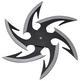Good day All,

Situation: I have col product name, datetime (!), product id. Those that are ProductName2, ProductName3, and ProductName4 we need to multiply the total quantitity by 12. The product labeled ProductName1, we need to multiply by 40.

To find the cumulative total, I used the below fomula and it worked just fine.

(COUNT(SELECT(@C:C;, (@AI:AI;="ProductName1")))*40)+(COUNT(SELECT(@C:C;, OR((@AI:AI;= "ProductName2"), (@AI:AI;="ProductName3"), (@AI:AI;="ProductName4"))))*12)/1000

Now, I need to get the cumulative total by month. For X-axis, I use:

DATERANGE(MIN(DATE(@J:J;, "dd-MMM-yyyy hh:mm:ss")),TODAY())

The question is how to build a formula for Series? I know that I need to use LOOKUP, GROUP and GROUPBY. But I am a little bit confused with how to handle the calculation within GROUPBY. Could you, please, advise?

LOOKUP(&'c5fd6550-3',GROUP(DATE_CONVERT(@J:J;, "dd-MMM-yyyy hh:mm:ss", "MMM yyyy")),GROUPBY(SELECT(@C:C;, (@AI:AI;="ProductName1"))*40)+SELECT(@C:C;, OR((@AI:AI;= "ProductName2"), (@AI:AI;="ProductName3"), (@AI:AI;="ProductName4"))*12))

#### 1 comment

•Janice Janczyn

Hi Eka,

Thank you for posting in our community!

First I'd like to suggest a more efficient formula to calculate your total product quantities.

(COUNT(SELECT(@C:C;, (@AI:AI;="ProductName1")))*40) +
(COUNT(SELECT(@C:C;, OR((@AI:AI;= "ProductName2"), (@AI:AI;="ProductName3"), (@AI:AI;="ProductName4"))))*12)    / 1000

Since you are only counting the values returned, you don't need to select them. Also, use the IN function to compare a value with multiple matches.

(COUNTIF( @AI:AI; = "ProductName1" ) * 40)  +
(COUNTIF( IN( @AI:AI;, ARRAY( "ProductName2", "ProductName3", "ProductName4") ) ) * 12)   / 1000

Note that you can simplify DATE & DATE_CONVERT functions by specifying just the date format since you aren't using the time values:

DATERANGE(MIN(DATE(@J:J;, "dd-MMM-yyyy")),TODAY())

Your series would be a bit different than the total product quantities formula since you want a daily breakdown, something like:

LOOKUP( &x-axis,
GROUP(DATE_CONVERT(@J:J;, "dd-MMM-yyyy", "MMM yyyy")),
GROUPBY(DATE_CONVERT(@J:J;, "dd-MMM-yyyy", "MMM yyyy"),
SWITCH( @AI:AI; , "ProductName1", 40,
"ProductName2", 12,
"ProductName3", 12,
"ProductName4", 12,
"_default_", 0 ) ) )  / 1000

If you need a cumulative result, wrap CUMULATIVE around the LOOKUP function.

I hope this is helpful. Happy dashboarding!

Janice